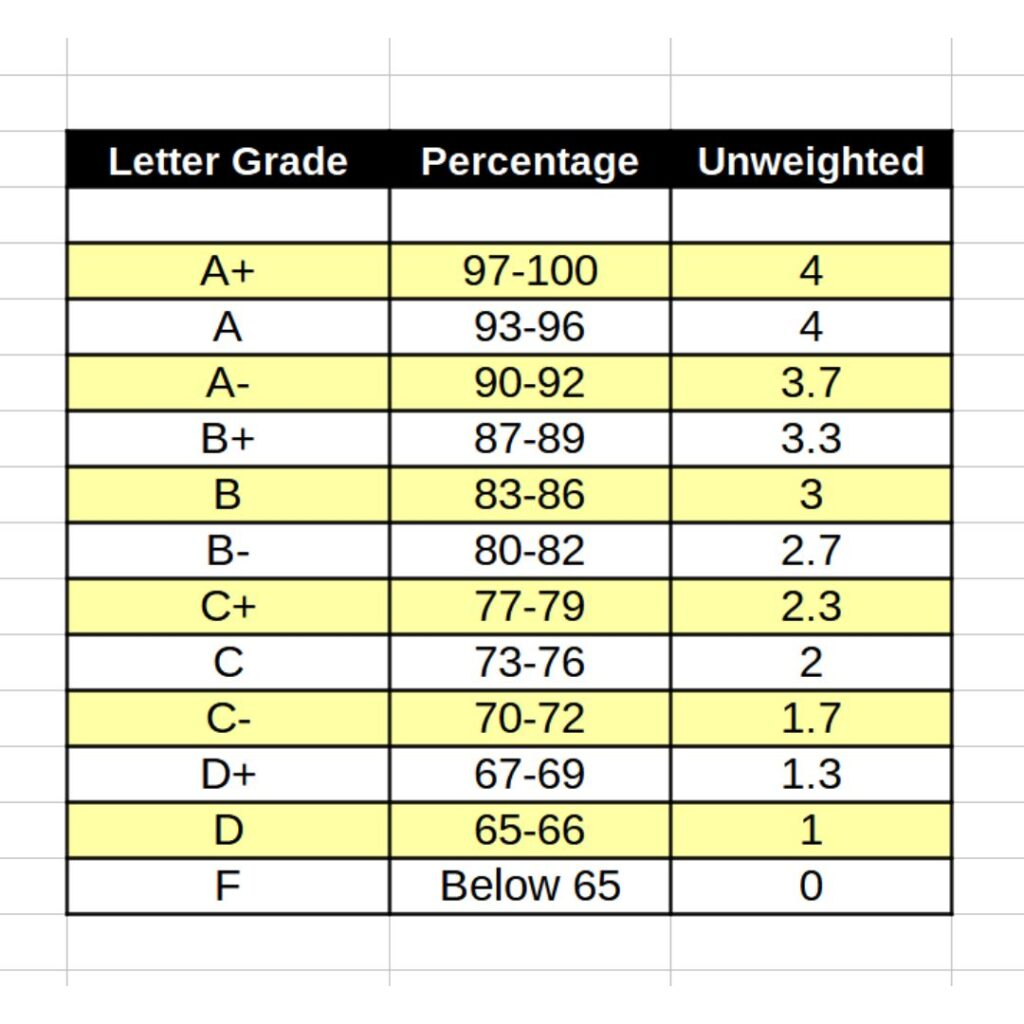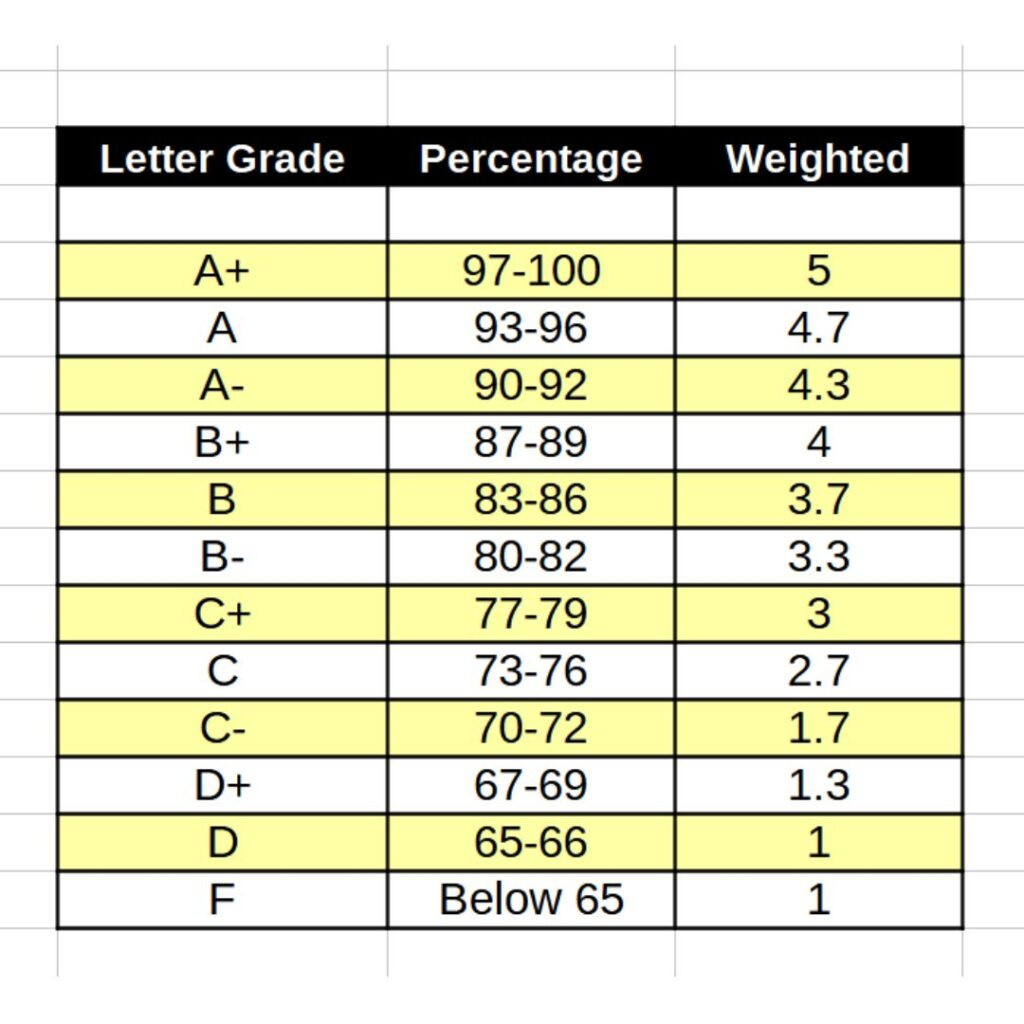# Which GPA do colleges look at | Curious to know ! |

GPA is the first prerequisite every college and university considers while shortlisting students for further admission screening.

You must be aware that GPA is categorized into two types: weighted GPA and unweighted GPA. In the high school transcript, one of them has been mentioned, and Now the query arises: Which GPA do colleges look at while shortlisting their admission list?

To get an answer to your query, you should first determine the difference between the weighted GPA and unweighted GPA with their calculation procedure, and then you will quickly understand how colleges consider one of them.

So here we begin.

## Unweighted GPA

Lorem ipsum dolor sit amet, consectetur adipiscing elit. Ut elit tellus, luctus nec ullamcorper mattis, pulvinar dapibus leo.

### How to calculate unweighted GPA

It’s a simple calculation. All the classes are reported on a 4.0-point scale for grades. For your convenience, you can see the chart below; it articulates each grade with its corresponding score.

With example, you can easily understand how to calculate an unweighted GPA if you got two As, two B and one C.

From Table [ A= 4.0, B =3.0 & C = 2.0 ]

• two A = ( 2 * 4.0 ) = 8

• two B= ( 2 * 3.0 ) = 6

• one C = (1 * 2.0) = 2Now, make the total of all outcomes. = 8 + 6 +2 = 16

And the total classes you take = 5

Here comes the outcomes = 16 ( total outcome of your grades) / 5 (Total classes taken)

It means your unweighted GPA is 3.2

## Weighted GPA

Most high schools in the US mention weighted GPA in students’ transcripts. The scale of weighted GPA varies as per class difficulty, while most high schools generally prefer 5.0.

In this GPA, students’ hard work towards the difficult classes has paid off, as some students take honour and AP classes.

### How to calculate weighted GPA

Suppose you have 2 AP Classes, One honour class and two regular classes.

• AP Physics (A+) = 5.0

• AP Computer Science (B+) = 4.0

• Maths Honors (A-) =4.3

• Chemistry (B-) = 3.3

• German (B) = 3.7Now, you have to take the total of all the scores. = = 5.0 + 4.0 + 4.3 + 3.3 + 3.7 = 20.3

And the total classes you intake = 5,

So the Weighted GPA = Total Score of the classes (20.3) / Total Class intake (5)

Here comes the weighted GPA is equal to 4.06

I understand that the calculation of weighted GPA is complex due to each class’s different GPA, but with the help of the example and table I have given, you can calculate easily.

## Which GPA do colleges look at

Until now, you have understood the meaning of both GPA-weighted and unweighted GPA well. As I am giving a scenario of how do colleges look at GPA,

Suppose a college gets the GPA of two students (A & B). Student A gains an unweighted GPA of 3.5 with an intake of challenging classes, while student B gets a weighted GPA 4.0 with less complicated classes. If the college prefers a GPA of student B, it will not be justice to student A, as it takes difficult classes.

The college uses its calculation formula to articulate equality among the students and recalculates all their GPAs. During discussions, some counsellors assume that college considers the subjects directly related to your course and calculates its unweighted GPA while separating non-academic ones.

In conclusion, each college has set its formula for shortlisting the students. Yes, you can consider first filtration as a GPA, but having a good GPA is not enough. Fulfilling other prerequisites is also essential, as each factor is vital in selecting your dream college or university.

### Last Recommendation

Don’t go too deep under this weighted GPA and unweighted GPA calculation. You should focus well on your studies without being burdened with AP classes. Take AP classes related to the college major you will take in the future, and if you are preparing for ACT and SAT scores, prepare well.